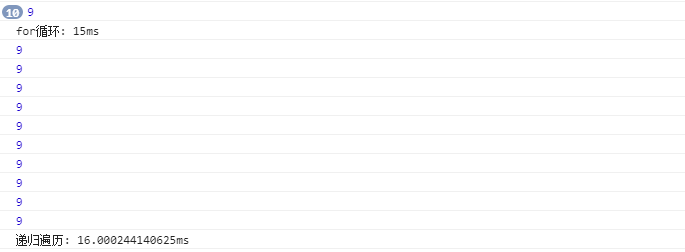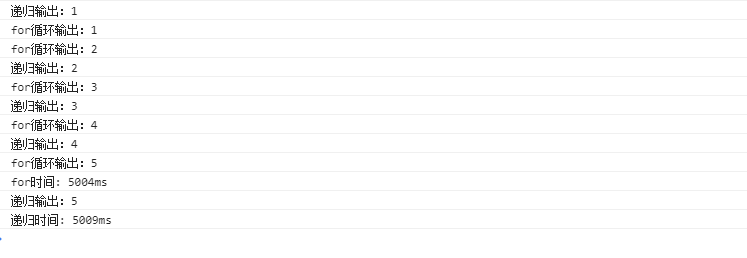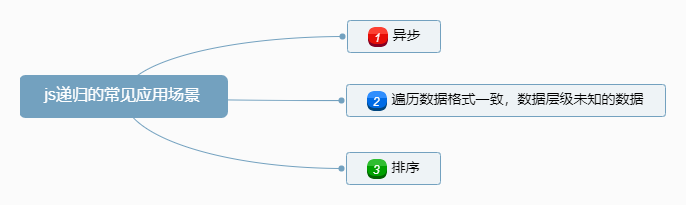# js递归遍历讲解

JavaScript的递归遍历会经常遇到，适当的运用递归遍历，可以提高代码性质量。

#### 1.某些时候递归能替换for循环

var arrList = [1,2,3,5,100,500,10000,10000,1000,10000002]
//for循环测试
function forTest(){
console.time("for循环")
for(let i in arrList){
console.log(((arrList[i] + arrList[i]) * 5 - arrList[i])/arrList[i])
}
console.timeEnd("for循环")
}
//递归遍历测试
function recursive() {
console.time("递归遍历")
const testFun = function (i) {
console.log(((arrList[i] + arrList[i]) * 5 - arrList[i])/arrList[i])
if(i == arrList.length - 1){
return
}
i++
testFun(i)
}
testFun(0)
console.timeEnd("递归遍历")
}
forTest()
recursive()#### 2.使用递归，可以轻松实现多级遍历

var data = [
{
name: "所有物品",
children: [
{
name: "水果",
children: [{name: "苹果", children: [{name: '青苹果'}, {name: '红苹果'}]}]
},
{
name: '主食',
children: [
{name: "米饭", children: [{name: '北方米饭'}, {name: '南方米饭'}]}
]
},
{
name: '生活用品',
children: [
{name: "电脑类", children: [{name: '联想电脑'}, {name: '苹果电脑'}]},
{name: "工具类", children: [{name: "锄头"}, {name: "锤子"}]},
{name: "生活用品", children: [{name: "洗发水"}, {name: "沐浴露"}]}
]
}
]
}]



//普通遍历实现
var forFunction = function () {
var str = ""
data.forEach(function(row){
row.children.forEach(function(row){
row.children.forEach(function(row){
row.children.forEach(function(row){
str += (row.name + ";")
})
})
})
})
console.log(str)
}
forFunction()
//输出：青苹果;红苹果;北方米饭;南方米饭;联想电脑;苹果电脑;锄头;锤子;洗发水;沐浴露;



//递归遍历实现
var recursiveFunction = function(){
var str = ''
const getStr = function(list){
list.forEach(function(row){
if(row.children){
getStr(row.children)
}else {
str += row.name + ";"
}
})
}
getStr(data)
console.log(str)
}
recursiveFunction()
//输出：青苹果;红苹果;北方米饭;南方米饭;联想电脑;苹果电脑;锄头;锤子;洗发水;沐浴露;



#### 3.递归遍历轻松实现多个异步结果的依次输出

//常规实现
var forTest = function () {
console.time("for时间")
for (let i = 0; i < 5; i++) {
setTimeout(function () {
console.log('for循环输出：' + (i + 1))
if(i+ 1 === 5){
console.timeEnd("for时间")
}
}, 1000 * (i + 1))
}
}
forTest()
//每隔一秒输出了1,2,3,4,5



//递归遍历实现
var recursiveTest = function(){
console.time("递归时间")
const test = function (i) {
setTimeout(function () {
i = i + 1
console.log('递归输出：' + i)
if(i < 5){
test(i)
}else {
console.timeEnd("递归时间")
}
}, 1000)
}
test(0)
}
recursiveTest()
//每隔一秒输出1,2,3,4,5#### 4.递归遍历实现排序

var quickSort = function(arr) {
if (arr.length <= 1) {//如果数组长度小于等于1无需判断直接返回即可
return arr;
}
var pivotIndex = Math.floor(arr.length / 2);//取基准点
var pivot = arr.splice(pivotIndex, 1);//取基准点的值,splice(index,1)函数可以返回数组中被删除的那个数
var left = [];//存放比基准点小的数组
var right = [];//存放比基准点大的数组
for (var i = 0; i < arr.length; i++){ //遍历数组，进行判断分配
if (arr[i] < pivot) {
left.push(arr[i]);//比基准点小的放在左边数组
} else {
right.push(arr[i]);//比基准点大的放在右边数组
}
}
//递归执行以上操作,对左右两个数组进行操作，直到数组长度为<=1；
return quickSort(left).concat([pivot], quickSort(right));
};

var arr = [14, 50, 80, 7, 2, 2, 11];
console.log(quickSort(arr));



#### 5.总结1. 很多时候可以用递归代替循环，可以理解为递归是一种特殊的循环，但通常情况下不推荐这样做。
2. 递归一般是在函数里面把函数自己给调用一遍，通过每次调用改变条件，来结束循环。
3. 递归在数据格式一致，在数据层级未知的情况下，比普通的遍历更有优势。
4. 递归在异步的时候，更容易理解，且更容易实现，因为可以在异步的回调里面，调用自己来实现每次都能拿到异步的结果再进行其他操作。
5. 递归实现的快速排序比普通遍历实现的排序效率更好。©️2019 CSDN 皮肤主题: 游动-白 设计师: 上身试试